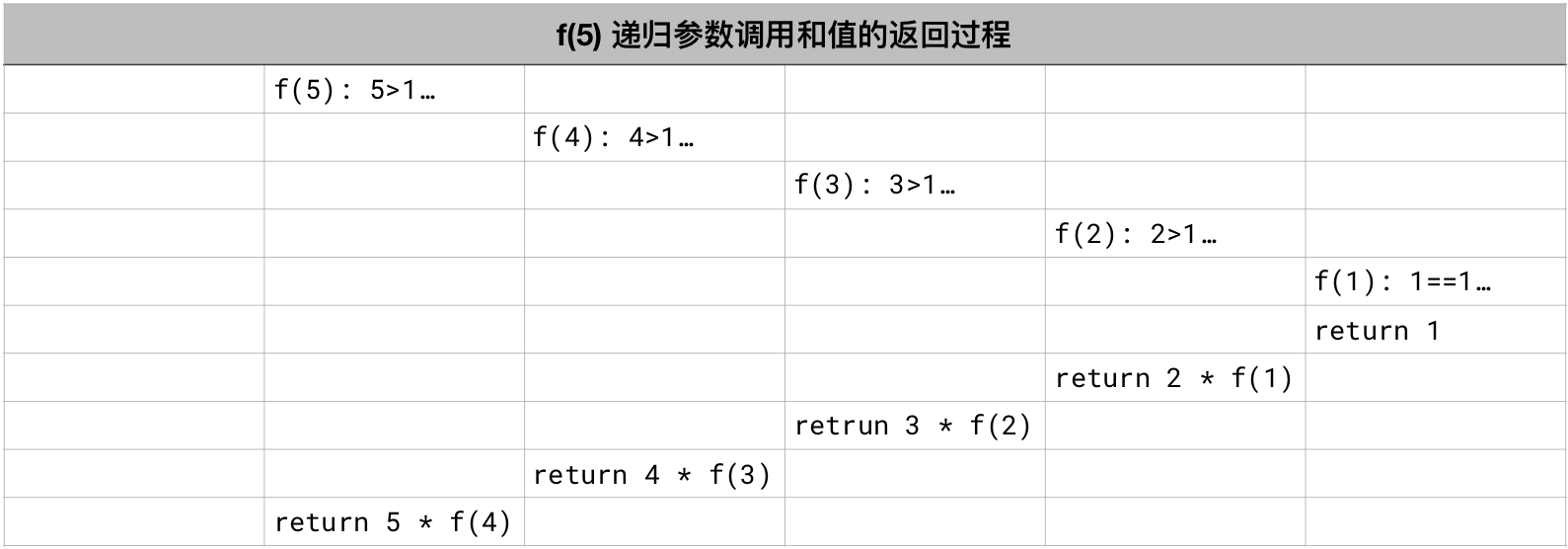Article Outline

递归函数

递归（Recursion）

• n! = n × (n-1) × (n-2)... × 1
• 即，n! = n × (n-1)!
• 且，n >= 1

def f(n):
if n == 1:
return 1
else:
return n * f(n-1)

print(f(5))
120

递归函数的执行过程• 因为 5 > 1，所以，在计算 n * f(n-1) 的时候要再次调用自己 f(4)；所以必须等待 f(4) 的值返回；
• 因为 4 > 1，所以，在计算 n * f(n-1) 的时候要再次调用自己 f(3)；所以必须等待 f(3) 的值返回；
• 因为 3 > 1，所以，在计算 n * f(n-1) 的时候要再次调用自己 f(2)；所以必须等待 f(2) 的值返回；
• 因为 2 > 1，所以，在计算 n * f(n-1) 的时候要再次调用自己 f(1)；所以必须等待 f(1) 的值返回；
• 因为 1 == 1，所以，这时候不会再次调用 f() 了，于是递归结束，开始返回，这次返回的是 1
• 下一步返回的是 2 * 1
• 下一步返回的是 3 * 2
• 下一步返回的是 4 * 6
• 下一步返回的是 5 * 24 —— 至此，外部调用 f(5) 的最终返回值是 120……

def f(n):
print('\tn =', n)
if n == 1:
print('Returning...')
print('\tn =', n, 'return:', 1)
return 1
else:
r = n * f(n-1)
print('\tn =', n, 'return:', r)
return r

print('Call f(5)...')
print('Get out of f(n), and f(5) =', f(5))
Call f(5)...
n = 5
n = 4
n = 3
n = 2
n = 1
Returning...
n = 1 return: 1
n = 2 return: 2
n = 3 return: 6
n = 4 return: 24
n = 5 return: 120
Get out of f(n), and f(5) = 120

递归的终点

def a_monk_telling_story():
print('山上有座庙，庙里有个和尚，和尚讲故事，他说…… ')
return a_monk_telling_story()

a_monk_telling_story()

• 一个是在梦里死掉；
• 一个是在梦里被 kicked 到……

• 入梦，in_dream()，是个递归函数；
• 入梦之后醒过来的条件有两个：
• 一个是在梦里死掉，dead is True
• 一个是在梦里被 kicked，kicked is True……

以上两个条件中任意一个被满足，就苏醒……

import random

def in_dream(day=0, dead=False, kicked=False):
dead = not random.randrange(0,10) # 1/10 probability to be dead
kicked = not random.randrange(0,10) # 1/10 probability to be kicked
day += 1

print((f"I slept {day} days, and was dead to wake up..."))
return day
elif kicked:
print(f"I slept {day} days, and was kicked to wake up...")
return day

return in_dream(day)

print('The in_dream() function returns:', in_dream())
dead: False kicked: False
dead: False kicked: False
dead: False kicked: False
dead: False kicked: False
dead: False kicked: False
dead: False kicked: False
dead: False kicked: False
dead: True kicked: True
I slept 8 days, and was dead to wake up...
The in_dream() function returns: 8

if condition:
pass

if condition is True:
pass

if condition == True:
pass

def x(n):
return n * x(n-1)
x(5)
---------------------------------------------------------------------------

RecursionError                            Traceback (most recent call last)

<ipython-input-3-daa4d33fb39b> in <module>
1 def x(n):
2     return n * x(n-1)
----> 3 x(5)

<ipython-input-3-daa4d33fb39b> in x(n)
1 def x(n):
----> 2     return n * x(n-1)
3 x(5)

... last 1 frames repeated, from the frame below ...

<ipython-input-3-daa4d33fb39b> in x(n)
1 def x(n):
----> 2     return n * x(n-1)
3 x(5)

RecursionError: maximum recursion depth exceeded

1. return 语句中返回的是自身的调用（或者是含有自身的表达式
2. 为了避免死循环，一定要有至少一个条件下返回的不再是自身调用……

变量的作用域

def factorial(n):
if n == 1:
return 1
else:
return n * factorial(n-1)

print(factorial(5))
120

• 在函数内部被赋值而后使用的，都是局部变量，它们的作用域是局部，无法被函数外的代码调用；
• 在所有函数之外被赋值而后开始使用的，是全局变量，它们的作用域是全局，在函数内外都可以被调用。

def factorial(n):
if n == 1:
return 1
else:
return n * factorial(n-1)

a = 5
b = factorial(a)   # a 并不会因此改变；
print(a, b)
a = factorial(a)   # 这是你主动为 a 再一次赋值……
print(a, b)
5 120
120 120

def factorial(n):
if n == 1:
return 1
else:
return n * factorial(n-1)

n = 5              # 这一次，这个变量名称是 n
m = factorial(n)   # n 并不会因此改变；
print(n, m)
5 120

m = factorial(n) 这一句中，nfactorial() 当做参数调用了，但无论函数内部如何操作，并不会改变变量 n 的值。

def factorial(x): # 在这个语句块中出现的变量，都是局部变量
if x == 1:
return 1
else:
return x * factorial(x-1)

n = 5           # 这一次，这个变量名称是 n
m = factorial(n)   # n 并不会因此改变；
print(n, m)
# 这个例子和之前再之前的示例代码有什么区别吗？
# 本质上没区别，就是变量名称换了而已……
5 120

递归函数三原则

1. 根据定义，递归函数必须在内部调用自己；
2. 必须设定一个退出条件；
3. 递归过程中必须能够逐步达到退出条件……

def teach_yourself(anything):
while not create():
learn()
practice()
return teach_yourself(another)

teach_yourself(coding)

思考与练习

https://introcs.cs.princeton.edu/java/23recursion/

1. ^ 参见 Stackoverflow 上的讨论：Boolean identity == True vs is True
2. ^ 关于阿西莫夫三铁律（Three Laws of Robotics）的类比，来自著名的 Python 教程，Think Python: How to Think Like a Computer Scientist